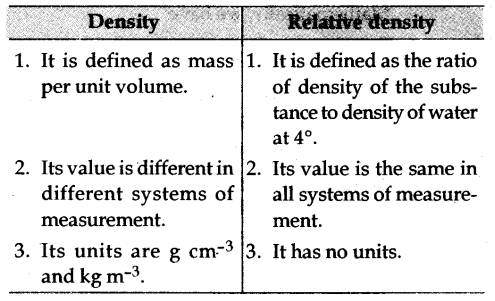# (a) Distinguish between density and relative density of a substance. (b) The relative density of silver is 10.8. If the density of water is 103 kg m^-3, find the density of silver

(a) Following are some of the important points of distinction between density and relative density :(b) Relative density of silver (RD) = 10.8
Density of water = 103 kg m^-3
Now, RD = Density of silver / Density of water
Therefore, we have
Density of silver = RD x Density of water
= 10.8 x 103 kg m^-3